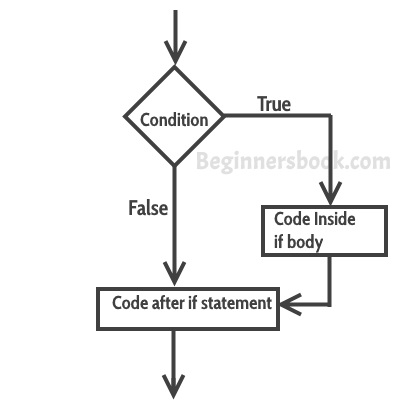If statement in C programming

When we need to execute a block of statements only when a given condition is true then we use if statement. In the next tutorial, we will learn C if..else, nested if..else and else..if.

C – If statement

Syntax of if statement:
The statements inside the body of “if” only execute if the given condition returns true. If the condition returns false then the statements inside “if” are skipped.

if (condition)
{
//Block of C statements here
//These statements will only execute if the condition is true
}

Flow Diagram of if statement